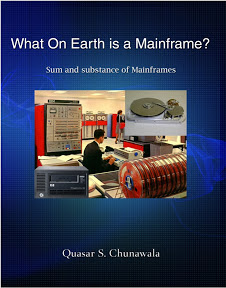Mainframes 360
The one stop destination for System Z professionals

## Monday, April 6, 2009

### JCL Pseudo-code - How to write output to Disk/Tape

 Q. How do we write Output to disk/tape in JCL? A. Unlike writing output to a dataset, disk/tape are direct Access(DASD) devices. Let us study the JCL that will write the output to the disk.

 //JOB1 JOB (A123),'QUASAR S', // CLASS=A,PRTY=6 //STEP1 EXEC PGM=PROGRAM1 //INPUT1 DD DSN=INFILE,DISP=SHR //DISK1 DD DSN=DISK.OUTPUT, // DISP=(NEW,CATLG,DELETE), // UNIT=DISK, // SPACE=(TRK,(1,1),RLSE), // DCB=(RECFM=FB,LRECL=40,BLKSIZE=400) //TAPE1 DD DSN=TAPE.OUTPUT, // DISP=(NEW,CATLG,DELETE), // UNIT=TAPE, // DCB=(RECFM=FB,LRECL=40,BLKSIZE=400)